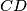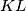### IMO Shortlist 1988 problem 6

Kvaliteta:
Avg: 0,0
Težina:
Avg: 0,0
In a given tedrahedron$ABCD$ let$K$ and$L$ be the centres of edges$AB$ and$CD$ respectively. Prove that every plane that contains the line$KL$ divides the tedrahedron into two parts of equal volume.
Izvor: Međunarodna matematička olimpijada, shortlist 1988